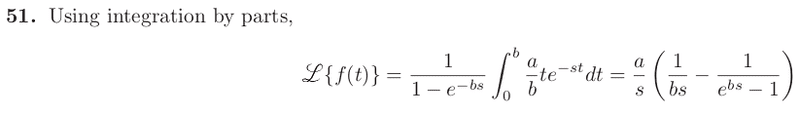# Laplace Transforms

Turion

Last edited:

Turion
The correct solution:Turion
Actually, I think the solutions manual is wrong. Please ignore this thread.

brmath
It would have helped if you stated Theorem 4.3.3. That said, we could try it without any special theorem. We have

##Lf = \int_0^{\infty} e^{-st}f(t)dt ##

which you can split up into the sum of integrals from (0,a) (a,2a) ... ([n-1]a,na) (na,t), where na < t < (n+1)a.

Each of these is easy enough to evaluate, but be careful to separate out the case where n is even and n is odd (why?). I'll bet you can factor some stuff out of the resulting sum.

Why don't you try this out? And why don't you quote theorem 4.3.3 -- maybe it's an easier way to do things.

Turion
It would have helped if you stated Theorem 4.3.3. That said, we could try it without any special theorem. We have

##Lf = \int_0^{\infty} e^{-st}f(t)dt ##

which you can split up into the sum of integrals from (0,a) (a,2a) ... ([n-1]a,na) (na,t), where na < t < (n+1)a.

Each of these is easy enough to evaluate, but be careful to separate out the case where n is even and n is odd (why?). I'll bet you can factor some stuff out of the resulting sum.

Why don't you try this out? And why don't you quote theorem 4.3.3 -- maybe it's an easier way to do things.